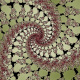## 3-torus

Here’s an equation for a a 3-torus I made:$(((x-4)^2+y^2+z^2+3^2-1^2)^2-4*3^2*((x-4)^2+y^2))*(((x+4)^2+y^2+z^2+3^2-1^2)^2-4*3^2*((x+4)^2+y^2))*((x^2+(y+6)^2+z^2+3^2-1^2)^2-4*3^2*(x^2+(y+6)^2))-5000000$

How did I make it? Consider describing it as a function  f(x,y,z)=0. This is called an implicit function. Since it’s the surface of zero values and zero times anything is zero, we superimpose functions by multiplying them. So if t1, t2, and t3 are tori, t1*t2*t3 is 3 tori. Now to smooth it out we subtract a number which causes the surface to move forward along something called a gradient.

Voila, a 3-trous!

Tags:

### 2 Responses to “3-torus”

1.3D printing of Mathematical Objects! « Christopher Olah's Blog Says:

[…] implicit plot of the 3-torus (see previous post) […]

2.Manipulation of Implicit Functions (With an Eye on CAD) « Christopher Olah's Blog Says:

[…] multiplication and subtraction — I used them a lot when I was younger (for example, making an equation for a 3-torus). The first trick is to realize that multiplying the functions for your implicit functions together […]